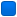###Author Topic: Compiler slow down  (Read 777 times)

####jonathan

• Member
•• Posts: 0##### Compiler slow down
« on: January 02, 2019, 02:02:48 am »
I am developing a signal processing app using Pelles C in a Windows 64bit console.  My code is processing streaming data and the main loop timing is critical.   In the loop I obtain a sin and cosine floating point values to use.  I have two approaches operating within the loop, one using a previously calculated lookup table and the other calculates it on the fly. Again both calculations are operating.  Both calculations produce identical values.  Yet when I use the values calculated on the fly the timing of the loop goes up by 80%!.  Here is the code segment:

//calculated on the fly
ptrst1=ptrst+j6+25000;
t8=*ptrst1;
ptrst1=(ptrst+j6);
t9=*ptrst1;
//lookup table
t6=*ptrs++;
t7=*ptrs++;
//   t6=t8;
//   t7=t9;

t8 is equal to t6 and t9 is equal to t7.  Yet when I take off the comment on last two lines the 80% slow down occurs.  I want to look at the assembly listing to see what is happening to the compiled code but I am unable to figure out how to get it from the compiler.  Whats going on?

####TimoVJL

• Global Moderator
• Member
••• Posts: 1882##### Re: Compiler slow down
« Reply #1 on: January 02, 2019, 08:41:29 am »
Compiler option /Tamd64-asm
Or use podump.exe with option /DISASM with object-file.
objconv